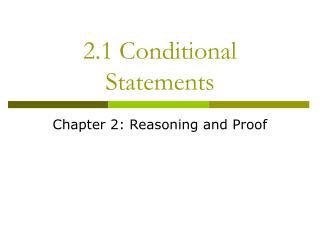DownloadDownload Presentation2.1 Conditional Statements

# 2.1 Conditional Statements

Download Presentation## 2.1 Conditional Statements

- - - - - - - - - - - - - - - - - - - - - - - - - - - E N D - - - - - - - - - - - - - - - - - - - - - - - - - - -
##### Presentation Transcript

1. 2.1 Conditional Statements Chapter 2: Reasoning and Proof

2. If –Then Statements Conditional: another name for an if-then statement “If you are not completely satisfied, then your money will be refunded.” Hypothesis: the part following the if Conclusion: the part following the then

3. Identifying the Hypothesis and Conclusion Identify the hypothesis and the conclusion of this conditional statement: “If today is the first day of fall, then the month is September.” Hypothesis: Conclusion: Today is the first day of fall The month is September

4. Writing a Conditional Write each sentence as a conditional: • A rectangle has four right angles. • A tiger is an animal. • An integer that ends with 0 is divisible by 5. • A square has four congruent sides. If a figure is a rectangle, then it has four right angles. If something is a tiger, then it is an animal. If an integer ends with 0, then it is divisible by 5. If a figure is a square, then it has four congruent sides.

5. Truth Value A conditional can have a truth value of true or false. To show that a conditional is true, show that every time the hypothesis is true, the conclusion is also true. To show that a conditional is false, you only need to find one counterexample.

6. Finding a Counterexample Show that this conditional is false by finding a counterexample: If it is February, then there are only 28 days in the month. Counterexample: There are 29 days in a leap year.

7. Finding a Counterexample Show that this conditional is false by finding a counterexample: If the name of a state contains the word New, then the state borders an ocean. Counterexample: New Mexico

8. Using a Venn Diagram Draw a Venn diagram to illustrate this conditional: “If you live in Chicago, then you live in Illinois.” Residents of Illinois Residents of Chicago

9. Using a Venn Diagram Draw a Venn diagram to illustrate this conditional: “If something is a Cocker Spaniel, then it is a dog.” Dogs Cocker Spaniels

10. Converse: switches the hypothesis and the conclusion Conditional: If two lines intersect to form right angles, then they are perpendicular. Converse: If two lines are perpendicular, then they intersect to form right angles.

11. Writing a converse Conditional: If two lines are not parallel and do not intersect, then they are skew. Converse: If two lines are skew, then they are not parallel and do not intersect.

12. Finding the Truth Value of a Converse Conditional: If a figure is a square, then it has four sides. Converse: If a figure has four sides, then it is a square. *Is this statement true? No, a rectangle also has four sides, so the converse is false. *Look in book, page 70, Summary box for Symbolic Form

13. Homework Pg 71 2 – 34 even, 64 – 67all